×

Search anything:Get this book -> Problems on Array: For Interviews and Competitive Programming

Reading time: 35 minutes | Coding time: 12 minutes

Linked List is a linear data structure similar to arrays but linked list elements are not stored at contiguous location; the elements are linked using pointers. There are three common variations of linked list, namely:

We will look into how Doubly Linked List(DLL) is useful and how it is implemented as well.Further, the advantages and disadvantages will be discussed

Doubly Linked List has the flexibility of traversing the list in both the ways i.e., forwad and backward unlike singly linked list where movement is restricted in forward direction only. Doubly Linked List contains an extra pointer to link the previous node which enables the backward traversing.

The following terminologies will be used for describing the various sections of a node in Doubly linked list :

• prev : The address of the previous node is saved here
• data : The data is stored here
• next : The address of the next node is saved here

A special pointer called Head pointer will be pointing to the first node of the list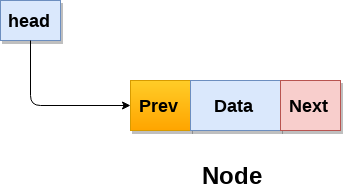A simple doubly linked list with 3 elements can be represented as follows(X represents NULL) :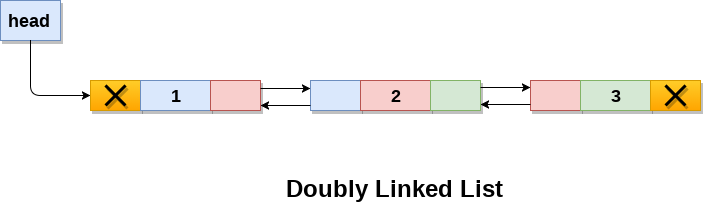### Basic Operations

Following are the basic operations that are done on any linked list :

• Insertion
• Deletion

#### Insertion :

We will consider the general case of insertion :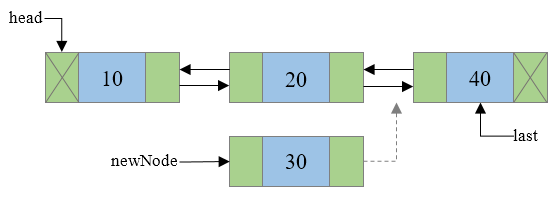Traverse to N-1 node in the list. Where N is the position to insert. Use a temp variable to point to N-1th node.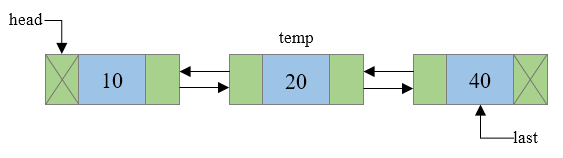Assign some data to the data field of the new_node.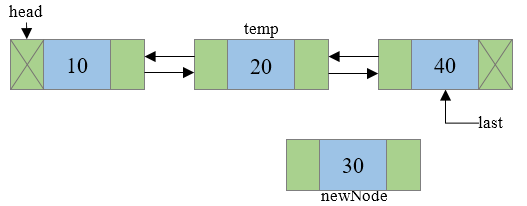Connect the next address field of new_node with the node pointed by next address field of temp node.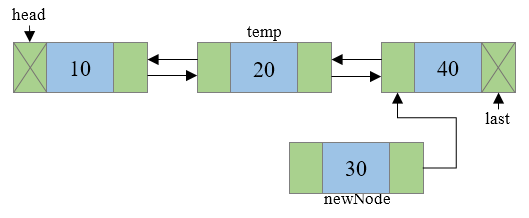Connect the previous address field of new_node with the temp node.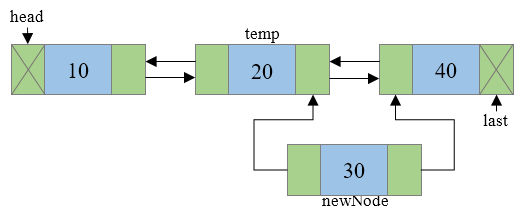Check if temp->next is not NULL then(i.e., if your temp is not the last element/node) connect the previous address field of node pointed by temp.next to new_node.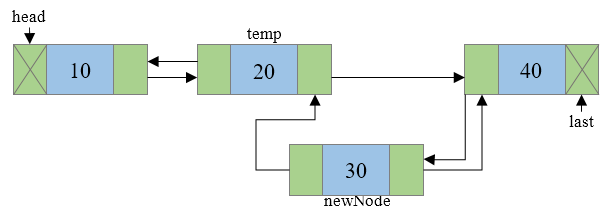Connect the next address field of temp node to new_node i.e. temp->next will now point to new_node.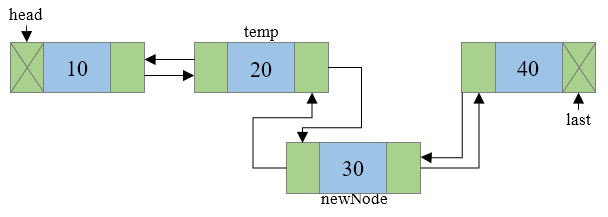The result would be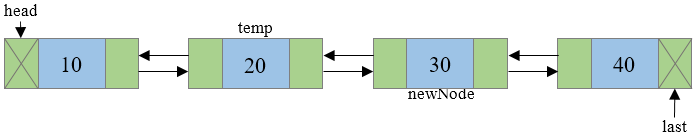### Deletion

We will consider the general case of deleting a node from doubly linked list

Let us delete the node 2 from the list. We will begin by traversing to that node (we will call it nth node for general case).Call it current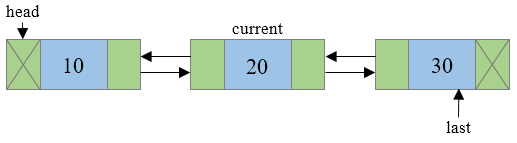Link the node behind current node with the node ahead of current node which can be implemented as current->prev->next = current->next.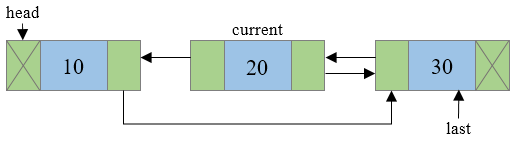If n+1th node is not NULL(i.e., if current node is not the last node) then link the n+1th node with n-1th node which can be implemented as current->next->prev = current->prev.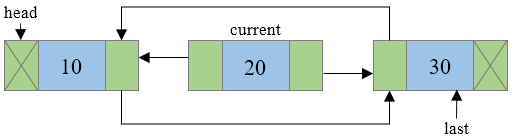Finally delete the current node from memory and you are done !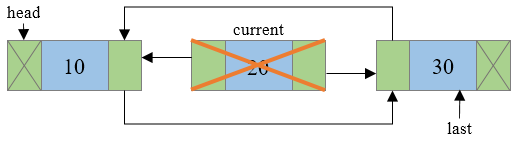Resultant list will be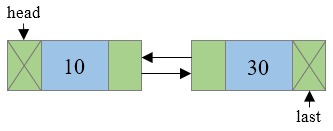### Complexity

1. Insertion
• Worst case time complexity: `Θ(n)`
• Average case time complexity: `Θ(1)`
• Best case time complexity: `Θ(1)`
• Space complexity: `Θ(1)`
2. Deletion
• Worst case time complexity: `Θ(n)`
• Average case time complexity: `Θ(1)`
• Best case time complexity: `Θ(1)`
• Space complexity: `Θ(1)`
3. Display
• Worst case time complexity: `Θ(n)`
• Average case time complexity: `Θ(n)`
• Best case time complexity: `Θ(n)`
• Space complexity: `Θ(1)`

### Implementations

``````#include<stdio.h>
#include<stdlib.h>
struct node
{
struct node *prev;
int data;
struct node *next;
void
insertAtFront (int value)
{
struct node *new_node = (struct node *) malloc (sizeof (struct node));
new_node->data = value;
{
new_node->next = NULL;
new_node->prev = NULL;
return;
}
temp->prev = new_node;
new_node->next = temp;
new_node->prev = NULL;
}
void
insertAfterValue (int value, int after_value)
{
struct node *new_node = (struct node *) malloc (sizeof (struct node));
new_node->data = value;
struct node *temp;
while (temp->data != after_value && temp->next != NULL)
{
temp = temp->next;
}
if (temp->next == NULL && temp->data != after_value)
{
printf ("Value not present\n");      return;
}
new_node->prev = temp;
new_node->next = temp->next;
if (temp->next != NULL)
temp->next->prev = new_node;
temp->next = new_node;
}
void
insertAtEnd (int value)
{
struct node *new_node = (struct node *) malloc (sizeof (struct node));
new_node->data = value;
{
new_node->next = NULL;
new_node->prev = NULL;
return;
}
struct node *temp;
while (temp->next != NULL)
{
temp = temp->next;
}
temp->next = new_node;
new_node->prev = temp;
new_node->next = NULL;
}
int
deleteAtFront ()
{
struct node *start_node;
int value;
start_node->next->prev = NULL;
value = start_node->data;  free (start_node);
return value;
}
int
deleteAtEnd ()
{
struct node *end_node;
int value;
while (end_node->next != NULL)
{
end_node = end_node->next;
}
end_node->prev->next = NULL;
value = end_node->data;
free (end_node);
return value;
}
int
deleteAfterValue (int after_value)
{
struct node *temp;
struct node *to_delete_node;
int value;
while (temp->data != after_value)
{
temp = temp->next;
}
to_delete_node = temp->next;
temp->next = to_delete_node->next;
if (temp->next != NULL)
to_delete_node->next->prev = temp;
value = to_delete_node->data;
free (to_delete_node);
return value;
}
void
display ()
{
struct node *temp;
while (temp->next != NULL)
{
printf ("%d->", temp->data);
temp = temp->next;
}
printf ("%d\n", temp->data);
}
int
main ()
{
int op, val, val2;
printf
("Enter 1: insertion at front\n2:
insertion at end\n3: insertion after a value\n4:
deletion at front\n5: deletion after a value\n6:
deletion at end\n7: display\n");
printf ("8: exit\n");
while (1)
{
scanf ("%d", &op);
switch (op)
{
case 1:
printf ("Enter the value\n");
scanf ("%d", &val);
insertAtFront (val);
break;
case 2:
printf ("Enter the value\n");
scanf ("%d", &val);
insertAtEnd (val);
break;
case 3:
printf ("Enter the value to be inserted\n");
scanf ("%d", &val);
printf
("Enter the value after which insertion should take place\n");
scanf ("%d", &val2);
insertAfterValue (val, val2);          break;
case 4:
val = deleteAtFront ();
printf ("Value deleted is : %d\n", val);
break;
case 5:
printf ("ENter the value after which deletion should take place\n");
scanf ("%d", &val2);
val = deleteAfterValue (val2);
printf ("Value deleted is : %d\n", val);
break;
case 6:
val = deleteAtEnd ();
printf ("Value deleted is : %d\n", val);
break;
case 7:
display ();
break;
case 8:
exit (0);
}
}
}
``````

### Applications

• It is used by browsers to implement backward and forward navigation of visited web pages i.e. back and forward button
• It is also used by various application to implement Undo and Redo functionality
• Applications that have a Most Recently Used list (a linked list of file names)

• A DLL can be traversed in both forward and backward direction.
• The delete operation in DLL is more efficient if pointer to the node to be deleted is given.
• We can quickly insert a new node before a given node.